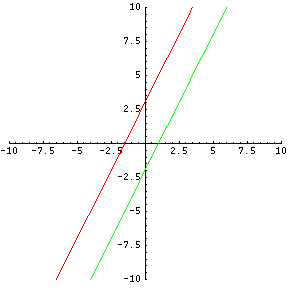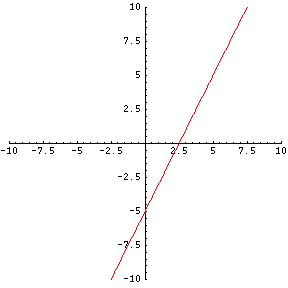# Solving Large Systems of Equations

To solve any system of linear equations, you will need to have at least the same number of independent equations as the number of variables. This can then be solved via the algebraic method or the algebraic elimination method. You can apply either method enough times to reduce each relation from a system of three or more equations with 3 or more variables to a system of 2 equations and two variables.

### Example

Solve for x, y and z:

$$2x - y + 6z = 1 \text{ (equation 1)}$$ $$x - y + z = 2 \text{ (equation 2)}$$ $$x + y + z = 1 \text{ (equation 3)}$$

### Steps to solve:

1) Solve equation 1 for y. After applying basic algebra skills, equation 1 becomes $$y = 2x + 6z - 1$$.

2) Plug the value of y, which is $$(2x + 6z -1)$$, into equations 2 and 3. Equation 2 becomes:

$$x - y + z = 2$$ $$x -(2x + 6z -1) + z = 2$$ $$x -2x - 6z + 1 = 2$$ $$-x - 6z = 2-1$$ $$-x -6z = 1$$

... and equation 3 becomes:

$$x + y + z = 1$$ $$x + 2x + 6z -1 + z = 1$$ $$3x + 7z -1 = 1$$ $$3x + 7z = -1+1$$ $$3x + 7z = 0$$

We now have come down to a system of two equations and two variables. Here are the two equations and two variables:

$$-x - 6z = 1 \text{ (new equation A) }$$ $$3x + 7z = 0 \text{ (new equation B) }$$

3) Solve equation A for x:

$$-x - 6z = 1$$ $$-x = 6z + 1$$ $$x = -6z - 1$$

4) Plug the value for x into equation B to solve for z:

$$3x + 7z = 0$$ $$3(-6z - 1) + 7z = 0$$ $$-18z - 3 + 7z = 0$$ $$-11z - 3 = 0$$ $$-11z = 3$$ $$z = \frac{-11}{3}$$

5) Now plug the value for z into equation A to find x:

$$-x - 6z = 1$$ $$-x -6\frac{-11}{3} = 1$$ $$-x + 22 = 1$$ $$-x = -22 + 1$$ $$-x = -21$$ $$x = 21$$

6) Lastly, go back to ANY of the original 3 equations to find the value of y by substituting what you found for x and z. I will select equation 3.

$$x + y + z = 1$$ $$21 + y - \frac{11}{3} = 1$$ $$\frac{52}{3} + y = 1$$ $$y = \frac{-52}{3} + 1$$ $$y = \frac{-49}{3}$$

$$x = 21, y = \frac{-49}{3}, z = \frac{-11}{3}$$

There are cases when NO solution to a system is possible. A solution to a system takes place where the graphs of the equations will cross or meet. Because of this, we can say that parallel lines (which have equal slopes) have NO solution because parallel lines never cross, touch or meet.

These two lines never meet, and thus have no intersection:It is also possible to face a question where BOTH equations share the SAME LINE on the xy-plane. For example, $$2r -s = 5$$ and $$4r - 2s = 10$$ can be reduced to $$s = 2r - 5$$. These two equations share the SAME LINE on the xy-plane. They are not independent, and there is no unique solution to the system.Originally by Mr. Feliz, © 2005